# 使用Sklearn学习朴素贝叶斯算法

1，sklearn中的贝叶斯分类器

1.1，高斯朴素贝叶斯GaussianNB

1.1.1，认识高斯朴素贝叶斯

1.1.2，参数说明

1.1.3，高斯朴素贝叶斯建模案例

1.1.4，探索高斯朴素贝叶斯擅长的数据集

1.1.5，探索贝叶斯：高斯朴素贝叶斯的拟合效果与运算速度

2，概率模型的评估指标

2.2.1，布里尔分数Brier Score

2.2.2，对数似然函数Log Loss

2.2.3，可靠性曲线Reliability Curve

2.2.4，预测概率的直方图

2.2.5，校准可靠性曲线

3，多项式朴素贝叶斯以及其变化

3.1，多项式朴素贝叶斯MultinomialNB

3.2，伯努利朴素贝叶斯BernoulliNB

3.3，探索贝叶斯：贝叶斯的样本不均衡问题

3.4，改进多项式朴素贝叶斯：补集朴素贝叶斯ComplementNB

# 1，sklearn中的贝叶斯分类器

Sklearn基于数据分布以及这些分布上的概率估计的改进，为我们提供了四个朴素贝叶斯的分类器。

 类 含义 naive_bayes.BernoulliNB 伯努利分布下的朴素贝叶斯 naive_bayes.GaussianNB 高斯分布下的朴素贝叶斯 naive_bayes.MultinomialNB 多项式分布下的朴素贝叶斯 naive_bayes.ComplementNB 补集朴素贝叶斯 linear_model.BayesianRidge 贝叶斯岭回归，在参数估计过程中使用贝叶斯回归技术来包括正则化参数

## 1.1，高斯朴素贝叶斯GaussianNB

### 1.1.1，认识高斯朴素贝叶斯

class sklearn.naive_bayes.GaussianNB (priors=None, var_smoothing=1e-09)

### 1.1.2，参数说明

 参数 含义 prior（先验概率） 可输入任何类数组结构，形状为（n_classes，） 表示类的先验概率。如果指定，则不根据数据调整先验，如果不指定，则自行根据数据计算先验概率。 var_smoothing 浮点数，可不填（默认值= 1e-9） 在估计方差时，为了追求估计的稳定性，将所有特征的方差中最大的方差以某个比例添加 到估计的方差中。这个比例，由var_smoothing参数控制。

### 1.1.3，高斯朴素贝叶斯建模案例

• 查看开发工具版本

%%cmd
pip install watermark #这是一个魔法命令
# 魔法命令必须写在一个cell的第一行

#在这里必须分开cell，魔法命令必须是一个cell的第一部分内容

%watermark -a "ruirui" -d -v -m -p numpy,pandas,matplotlib,scipy,sklearn
• 建模
import numpy as np
import matplotlib.pyplot as plt
from sklearn.naive_bayes import GaussianNB
from sklearn.model_selection import train_test_split

# 导入数据集

X=digits.data
y=digits.target

# 划分测试集和训练数据集,划分后，训练数据1257个样本，测试数据集540个样本
xtrain,xtest,ytrain,ytest=train_test_split(X,y,test_size=0.3,random_state=0)

# 查看标签种类
np.unique(ytrain)

# 实例化模型并且训练模型，其中fit()过程就是在计算概率的过程
gnb=GaussianNB().fit(xtrain,ytrain)

# score()接口对于我们的分类型算法，返回预测的精确性，也就是accuracy，使用测试数据集测试
acc_score=gnb.score(xtest,ytest)

# 返回所有样本对应的类别，这里的样本标签是用我们下面得到的概率中，
# 选取每一个样本中概率最大的作为此样本的标签
y_pred=gnb.predict(xtest)

# 查看我们的概率结果
yprob=gnb.predict_proba(xtest)
# 可以看到，返回的结果是540*10的二维矩阵，其中应为分类有10个，所以一共返回10列概率
# 取其中概率最大的哪一个分类作为最后的分类结果，并且每一行的概率之和是1

#注意，ROC曲线是不能用于多分类的。多分类状况下最佳的模型评估指标是混淆矩阵和整体的准确度
from sklearn.metrics import confusion_matrix as CM
CM(ytest,y_pred)

### 1.1.4，探索高斯朴素贝叶斯擅长的数据集

import numpy as np
import matplotlib.pyplot as plt
from matplotlib.colors import ListedColormap
from sklearn.model_selection import train_test_split
from sklearn.preprocessing import StandardScaler
from sklearn.datasets import make_moons, make_circles, make_classification
from sklearn.naive_bayes import GaussianNB,MultinomialNB,BernoulliNB,ComplementNB

h = .02
# 模型的名字
names = ["Multinomial","Gaussian","Bernoulli","Complement"]
# 创建我们的模型对象
classifiers = [MultinomialNB(),GaussianNB(),BernoulliNB(),ComplementNB()]
# 创建分类数据集
X, y = make_classification(n_features=2, n_redundant=0, n_informative=2,
random_state=1, n_clusters_per_class=1)
# 月亮刑数据
rng = np.random.RandomState(2)
X += 2 * rng.uniform(size=X.shape)
linearly_separable = (X, y)

datasets = [make_moons(noise=0.3, random_state=0),
make_circles(noise=0.2, factor=0.5, random_state=1),
linearly_separable
]
# 创建画布
figure = plt.figure(figsize=(6, 9))
i = 1

for ds_index, ds in enumerate(datasets):
X, y = ds
#     标准化数据集
X = StandardScaler().fit_transform(X)
X_train, X_test, y_train, y_test = train_test_split(X, y, test_size=.4,random_state=42)
#     对画布画网格线
x1_min, x1_max = X[:, 0].min() - .5, X[:, 0].max() + .5
x2_min, x2_max = X[:, 1].min() - .5, X[:, 1].max() + .5
array1,array2 = np.meshgrid(np.arange(x1_min, x1_max, 0.2),
np.arange(x2_min, x2_max, 0.2))
cm = plt.cm.RdBu
cm_bright = ListedColormap(['#FF0000', '#0000FF'])
ax = plt.subplot(len(datasets), 2, i)
if ds_index == 0:
ax.set_title("Input data")
ax.scatter(X_train[:, 0], X_train[:, 1], c=y_train,
cmap=cm_bright,edgecolors='k')
ax.scatter(X_test[:, 0], X_test[:, 1], c=y_test,
cmap=cm_bright, alpha=0.6,edgecolors='k')
ax.set_xlim(array1.min(), array1.max())
ax.set_ylim(array2.min(), array2.max())
ax.set_xticks(())
ax.set_yticks(())
i += 1
ax = plt.subplot(len(datasets),2,i)
clf = GaussianNB().fit(X_train, y_train)
score = clf.score(X_test, y_test)
Z = clf.predict_proba(np.c_[array1.ravel(),array2.ravel()])[:, 1]
Z = Z.reshape(array1.shape)
ax.contourf(array1, array2, Z, cmap=cm, alpha=.8)
ax.scatter(X_train[:, 0], X_train[:, 1], c=y_train, cmap=cm_bright,
edgecolors='k')
ax.scatter(X_test[:, 0], X_test[:, 1], c=y_test, cmap=cm_bright,
edgecolors='k', alpha=0.6)
ax.set_xlim(array1.min(), array1.max())
ax.set_ylim(array2.min(), array2.max())
ax.set_xticks(())
ax.set_yticks(())
if ds_index == 0:
ax.set_title("Gaussian Bayes")
ax.text(array1.max() - .3, array2.min() + .3, ('{:.1f}%'.format(score*100)),
size=15, horizontalalignment='right')
i += 1
plt.tight_layout()
plt.show()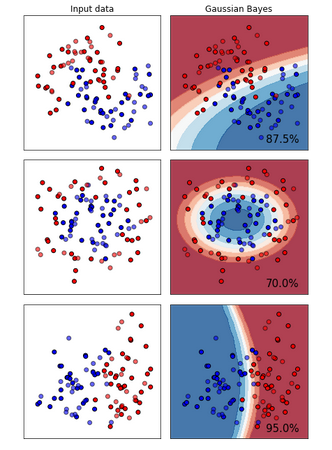### 1.1.5，探索贝叶斯：高斯朴素贝叶斯的拟合效果与运算速度

• 导入需要的模块
import numpy as np
import matplotlib.pyplot as plt
from sklearn.naive_bayes import GaussianNB
from sklearn.svm import SVC
from sklearn.ensemble import RandomForestClassifier as RFC
from sklearn.tree import DecisionTreeClassifier as DTC
from sklearn.linear_model import LogisticRegression as LR
from sklearn.model_selection import learning_curve
from sklearn.model_selection import ShuffleSplit
from time import time
import datetime
• 交叉验证的模式
datas=load_digits()
X=datas.data
y=datas.target
clf=GaussianNB()
# 交叉验证的模式
# 这里使用的训练集数据比例是默认的，5次
cv = ShuffleSplit(n_splits=50# 标示把数据分成多少份
, test_size=0.2# 其中有20%数据作为测试集
, random_state=0)# 在交叉验证的时候进行的随机抽样的模式
# 把数据分50分，其中20%作为测试集，50此测试，每一次都用20%的数据集作为测试
learning_curve(clf# 标示分类器
, X, y# 特征矩阵和标签
,cv=cv# 设置交叉验证的模式
,n_jobs=4)# 每一次运行可以使用的线程数目

train_sizes, train_scores, test_scores = learning_curve(clf, X, y
,cv=cv,n_jobs=3)

train_sizes
# 训练集里面数据的比例，array([ 143,  467,  790, 1113, 1437])
# 分成5次训练，训练数据样本逐渐增加，每一次划分，都把数据集划分为50分
train_scores.shape #(5, 50)

test_scores.shape# (5, 50)

# 画曲线的函数
# 第一步：找出每一个图的横纵坐标

def plot_learning_curve(estimator,title, X, y,# estimator标示使用的分类器
ax, #选择子图
ylim=None, #设置纵坐标的取值范围
cv=None, #交叉验证
n_jobs=None #设定索要使用的线程
):
#     此函数会返回图像的横纵坐标，但是不会画出图像
train_sizes, train_scores, test_scores = learning_curve(estimator, X, y
,cv=cv,n_jobs=n_jobs)
# train_sizes每一次划分训练集和测试集后，训练集上面样本的数量
# train_scores得到的是训练集上面的分数
# test_scores得到最后的测试分数
ax.set_title(title)
if ylim is not None:# 设置y坐标的量纲形式一样，也就是把多个子图的y坐标设置为一样
ax.set_ylim(*ylim)
ax.set_xlabel("Training examples")
ax.set_ylabel("Score")
ax.grid() #显示网格作为背景，不是必须
ax.plot(train_sizes, np.mean(train_scores, axis=1), 'o-'
, color="r",label="Training score")# 画训练数据集的图像
ax.plot(train_sizes, np.mean(test_scores, axis=1), 'o-'
, color="g",label="Test score")# 画出测试集图像
ax.legend(loc="best")
return ax
• 绘制曲线
title = ["Naive Bayes","DecisionTree","SVM, RBF kernel","RandomForest","Logistic"]
model = [GaussianNB(),DTC(),SVC(gamma=0.001)
,RFC(n_estimators=50),LR(C=.1,solver="lbfgs")]# 实例化我们的模型算法
fig, axes = plt.subplots(1,5,figsize=(30,6))# 设置子图的个数
for ind,title_,estimator in zip(range(len(title)),title,model):
times = time()
plot_learning_curve(estimator, title_, X, y,
ax=axes[ind], ylim = [0.7, 1.05],n_jobs=4, cv=cv)
print("{}:{}".format(title_,datetime.datetime.fromtimestamp(time()-
times).strftime("%M:%S:%f")))
plt.show()
# 图像的横坐标标示训练样本的个数
# 如果数据集的维度很高，我们就使用贝叶斯算法，对于一般的数据集我们首选逻辑回归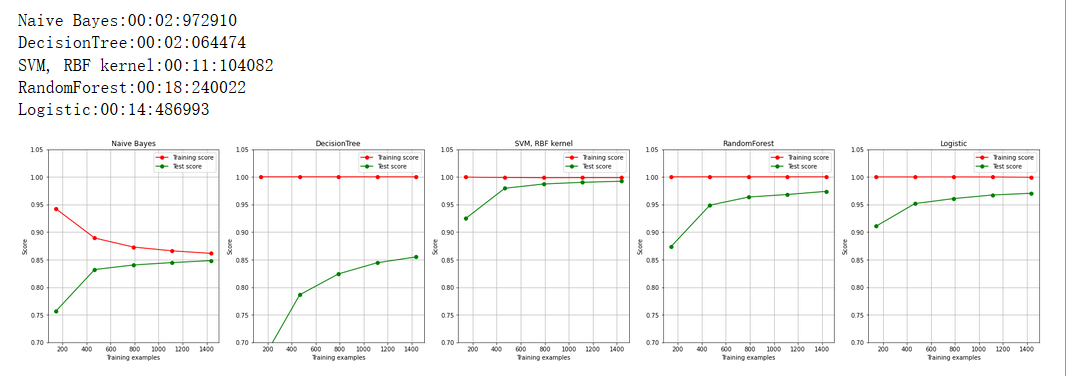## 2，概率模型的评估指标

### 2.2.1，布里尔分数Brier Score

，其中N是样本数量，为朴素贝叶斯预测出的概率， 是样本所对应的真实结果，只能取到0或者1，如果事件发生则为1，如果不发生则为0。这个指标衡量了我们的概率距离真实标签结果的差异，其实看起来非常像是均方误差。布里尔分数的范围是从0到1，分数越高则预测结果越差劲，校准程度越差，因此布里尔分数越接近0越好。由于它的本质也是在衡量一种损失，所以在sklearn当中，布里尔得分被命名为brier_score_loss。我们可以从模块metrics中导入这个分数来衡量我们的模型评估结果：

from sklearn.metrics import brier_score_loss
#注意，第一个参数是真实标签，第二个参数是预测出的概率值
#在二分类情况下，接口predict_proba会返回两列，但SVC的接口decision_function却只会返回一列
#要随时注意，使用了怎样的概率分类器，以辨别查找置信度的接口，以及这些接口的结构
brier_score_loss(Ytest, prob[:,1], pos_label=1)
#我们的pos_label与prob中的索引一致，就可以查看这个类别下的布里尔分数是多少

brier_score_loss(Ytest,prob[:,8],pos_label=8)

# 创建模型并且拟合数据
logi = LR(C=1., solver='lbfgs',max_iter=5000,multi_class="auto").fit(xtrain,ytrain)
svc = SVC(kernel = "linear",gamma=1).fit(xtrain,ytrain)

# 逻辑回归的布里尔分数
brier_score_loss(ytest,logi.predict_proba(xtest)[:,1],pos_label=1)

#由于SVC的置信度并不是概率，为了可比性，我们需要将SVC的置信度“距离”归一化，压缩到[0,1]之间
svc_prob = (svc.decision_function(Xtest) -
svc.decision_function(Xtest).min())/(svc.decision_function(Xtest).max() -
svc.decision_function(Xtest).min())
brier_score_loss(Ytest,svc_prob[:,1],pos_label=1)

# 数据可视化
import pandas as pd
name = ["Bayes","Logistic","SVC"]
color = ["red","black","orange"]
df = pd.DataFrame(index=range(10),columns=name)
for i in range(10):
df.loc[i,name] = brier_score_loss(Ytest,prob[:,i],pos_label=i)
df.loc[i,name] = brier_score_loss(Ytest,logi.predict_proba(Xtest)
[:,i],pos_label=i)
df.loc[i,name] = brier_score_loss(Ytest,svc_prob[:,i],pos_label=i)
for i in range(df.shape):
plt.plot(range(10),df.iloc[:,i],c=color[i])
plt.legend()
plt.show()
df

### 2.2.2，对数似然函数Log Loss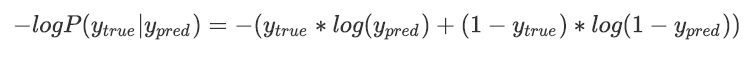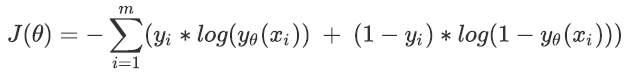# 似然对数损失
from sklearn.metrics import log_loss
# 似然损失是可以衡量多分类的

log_loss(ytest,yprob)

# 第一个参数是真实的标签，第二个参数是概率值
log_loss(ytest,logi.predict_proba(xtest))

log_loss(ytest,svc_prob)

 需求 优先使用对数似然 优先使用布里尔分数 衡量模型 要对比多个模型，或者衡量模型的不同变化 衡量单一模型的表现 可解释性 机器学习和深度学习之间的行家交流，学术论文 商业报告，老板开会，业务模型的衡量 最优化指向 逻辑回归，SVC 朴素贝叶斯 数学问题 概率只能无限接近于0或1，无法取到0或1 概率可以取到0或1，比如树，随机森林

### 2.2.3，可靠性曲线Reliability Curve

• 导入需要的模块
# 可靠性曲线
import numpy as np
import matplotlib.pyplot as plt
from sklearn.datasets import make_classification as mc
from sklearn.naive_bayes import GaussianNB
from sklearn.svm import SVC
from sklearn.linear_model import LogisticRegression as LR
from sklearn.metrics import brier_score_loss
from sklearn.model_selection import train_test_split
• 创建数据集
# 创建数据集
X, y = mc(n_samples=100000,n_features=20 #总共20个特征
,n_classes=2 #标签为2分类
,n_informative=2 #其中两个代表较多信息
,n_redundant=10 #10个都是冗余特征
,random_state=42)
xtrain,xtest,ytrain,ytest=train_test_split(X,y,test_size=0.99,random_state=0)
• 建立模型
gns=GaussianNB()
gns=gns.fit(xtrain,ytrain)
# 导出分类的结果
y_pred=gns.predict(xtest)
# 导出预测概率
y_prob=gns.predict_proba(xtest)[:,1]
• 绘制图像
y_prob.shape
# 我们的预测概率，作为横坐标
# ytest 我们的真实标签，作为纵坐标

import pandas as pd
#在我们的横纵表坐标上，概率是由顺序的（由小到大），为了让图形规整一些，我们要先对预测概率和真实标签按照预测
# 概率进行一个排序，这一点我们通过DataFrame来实现
df=pd.DataFrame({"ytrue":ytest[:500],"probability":y_prob[:500]})

# 对df中的数据进行排序
df=df.sort_values(by="probability")
# 现在恢复我们的索引
df.index=range(df.shape)

#紧接着我们就可以画图了
fig = plt.figure()
ax1 = plt.subplot()
ax1.plot([0, 1], [0, 1], "k:", label="Perfectly calibrated") #得做一条对角线来对比呀
ax1.plot(df["probability"],df["ytrue"],"s-",label="%s (%1.3f)" % ("Bayes", 3))
ax1.set_ylabel("True label")
ax1.set_xlabel("predcited probability")
ax1.set_ylim([-0.05, 1.05])
ax1.legend()
plt.show()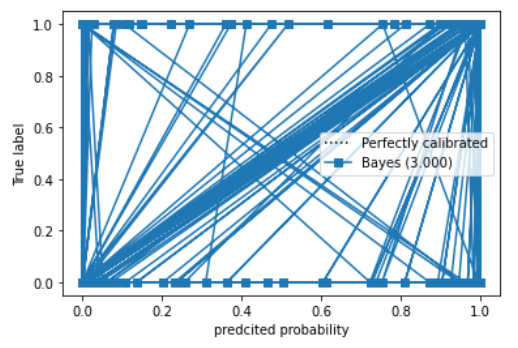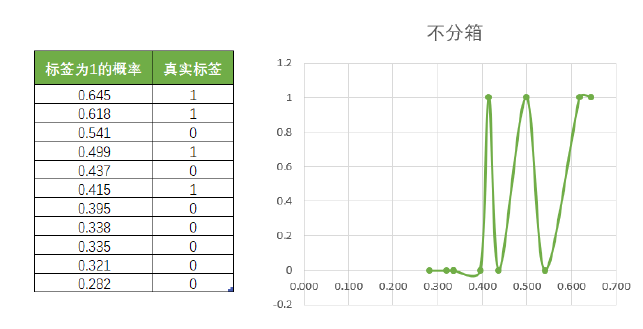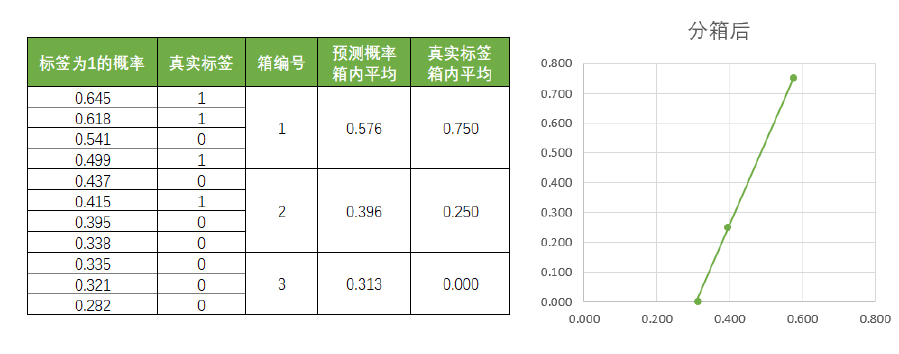参数 含义 y_true 真实标签 y_prob 预测返回的，正类别下的概率值或置信度 normalize 布尔值，默认False 是否将y_prob中输入的内容归一化到[0,1]之间，比如说，当y_prob并不是真正的概率的时候可使用。如果这是为True，则会将y_prob中最小的值归一化为0，最大值归一化为1。 n_bins 整数值，表示分箱的个数。如果箱数很大，则需要更多的数据 返回 含义 trueproba 可靠性曲线的纵坐标，结构为(n_bins, )，是每个箱子中少数类(Y=1)的占比 predproba 可靠性曲线的横坐标，结构为(n_bins, )，是每个箱子中概率的均值
• 重新绘制上面的可靠性曲线
from sklearn.calibration import calibration_curve

# 分成10箱，ytest代表是真实标签，y_prob标示返回的概率
trueproba,predproba=calibration_curve(ytest,y_prob,n_bins=10)
# predproba代表每一个箱子中概率的均值
# trueproba代表每一个箱子中少数类的占比，均值
trueproba
# 我们要求分10个箱子，所以会返回10个数字

#紧接着我们就可以画图了
fig = plt.figure()
ax1 = plt.subplot()
ax1.plot([0, 1], [0, 1], "k:", label="Perfectly calibrated") #得做一条对角线来对比呀
ax1.plot(predproba,trueproba,"s-",label="%s (%1.3f)" % ("Bayes", 10))# 10代表是分10个箱子
ax1.set_ylabel("True label")
ax1.set_xlabel("predcited probability")
ax1.set_ylim([-0.05, 1.05])
ax1.legend()
plt.show()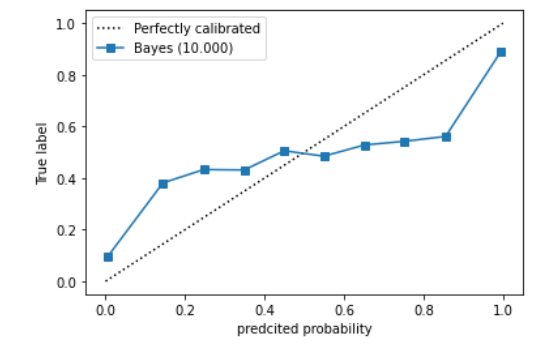# 探索不同箱子的个数对模型的影响
fig,axes=plt.subplots(1,3,figsize=(18,4))
for ind,i in enumerate([3,10,100]):
ax1=axes[ind]
trueproba,predproba=calibration_curve(ytest,y_prob,n_bins=i)
ax1.plot([0, 1], [0, 1], "k:", label="Perfectly calibrated") #得做一条对角线来对比呀
ax1.plot(predproba,trueproba,"s-",label="%s (%1.3f)" % ("Bayes", i))
ax1.set_ylabel("True label")
ax1.set_xlabel("predcited probability")
ax1.set_ylim([-0.05, 1.05])
ax1.legend()
plt.show()
# 当n=100的时候，明显是过拟合
# 我们绘制出的图像，越接近y=x这条直线，越好

### 2.2.4，预测概率的直方图

# 直方图的操作
fig,ax2=plt.subplots(figsize=(8,6))
name = ["GaussianBayes","Logistic","SVC"]
gnb = GaussianNB()
logi = LR(C=1., solver='lbfgs',max_iter=3000,multi_class="auto")
svc = SVC(kernel = "linear",gamma=1)
for clf, name_ in zip([gnb,logi,svc],name):
clf.fit(xtrain,ytrain)
y_pred = clf.predict(xtest)
#hasattr(obj,name)：查看一个类obj中是否存在名字为name的接口，存在则返回True
if hasattr(clf, "predict_proba"):
prob_pos = clf.predict_proba(xtest)[:,1]
else: # use decision function
prob_pos = clf.decision_function(xtest)
prob_pos = (prob_pos - prob_pos.min()) / (prob_pos.max() - prob_pos.min())
ax2.hist(prob_pos
,bins=10
,label=name_
,histtype="step" #设置直方图为透明
,lw=2 #设置直方图每个柱子描边的粗细
)
ax2.set_ylabel("Distribution of probability")
ax2.set_xlabel("Mean predicted probability")
ax2.set_xlim([-0.05, 1.05])
ax2.set_xticks([0,0.1,0.2,0.3,0.4,0.5,0.6,0.7,0.8,0.9,1])
ax2.legend(loc=9)
plt.show()

### 2.2.5，校准可靠性曲线

class sklearn.calibration.CalibratedClassifierCV (base_estimator=None, method=’sigmoid’, cv=’warn’)

• base_estimator：需要校准其输出决策功能的分类器，必须存在predict_proba或decision_function接口。 如果参数cv = prefit，分类
器必须已经拟合数据完毕。
• cv:整数，确定交叉验证的策略。可能输入是：
• None，表示使用默认的3折交叉验证
• 任意整数，指定折数,对于输入整数和None的情况下来说，如果是二分类，则自动使用类sklearn.model_selection.StratifiedKFold进行折数分割。如果y是连续型变量，则使用sklearn.model_selection.KFold进行分割。
• 已经使用其他类建好的交叉验证模式或生成器cv
• 可迭代的，已经分割完毕的测试集和训练集索引数组
• 输入"prefit"，则假设已经在分类器上拟合完毕数据。在这种模式下，使用者必须手动确定用来拟合分类器的数据与即将倍校准的数据没有交集
• method:进行概率校准的方法，可输入"sigmoid"或者"isotonic"
• 输入'sigmoid'，使用基于Platt的Sigmoid模型来进行校准
• 输入'isotonic'，使用等渗回归来进行校准

• 函数包装

def plot_calib(models,name,Xtrain,Xtest,Ytrain,Ytest,n_bins=10):
import matplotlib.pyplot as plt
from sklearn.metrics import brier_score_loss
from sklearn.calibration import calibration_curve
fig, (ax1, ax2) = plt.subplots(1, 2,figsize=(20,6))
ax1.plot([0, 1], [0, 1], "k:", label="Perfectly calibrated")# 画出y=x的直线
for clf, name_ in zip(models,name):
clf.fit(xtrain,ytrain)
y_pred = clf.predict(Xtest)
#hasattr(obj,name)：查看一个类obj中是否存在名字为name的接口，存在则返回True
if hasattr(clf, "predict_proba"):
prob_pos = clf.predict_proba(Xtest)[:,1]
else: # use decision function
prob_pos = clf.decision_function(Xtest)
prob_pos = (prob_pos - prob_pos.min()) / (prob_pos.max() - prob_pos.min())
#返回布里尔分数
clf_score = brier_score_loss(Ytest, prob_pos, pos_label=y.max())
trueproba, predproba = calibration_curve(Ytest, prob_pos,n_bins=n_bins)
ax1.plot(predproba, trueproba,"s-",label="%s (%1.3f)" % (name_, clf_score))
ax2.hist(prob_pos, range=(0, 1), bins=n_bins, label=name_,histtype="step",lw=2)
ax2.set_ylabel("Distribution of probability")
ax2.set_xlabel("Mean predicted probability")
ax2.set_xlim([-0.05, 1.05])
ax2.legend(loc=9)
ax2.set_title("Distribution of probablity")
ax1.set_ylabel("True probability for class 1")
ax1.set_xlabel("Mean predcited probability")
ax1.set_ylim([-0.05, 1.05])
ax1.legend()
ax1.set_title('Calibration plots(reliability curve)')
plt.show()

from sklearn.calibration import CalibratedClassifierCV
name = ["GaussianBayes","Logistic","Bayes+isotonic","Bayes+sigmoid"]
gnb = GaussianNB()
models = [gnb
,LR(C=1., solver='lbfgs',max_iter=3000,multi_class="auto")
#定义两种校准方式
,CalibratedClassifierCV(gnb, cv=2, method='isotonic')
,CalibratedClassifierCV(gnb, cv=2, method='sigmoid')]

plot_calib(models,name,xtrain,xtest,ytrain,ytest)

• 基于校准结果查看精确性的变化
gnb = GaussianNB().fit(Xtrain,Ytrain)
gnb.score(Xtest,Ytest)
brier_score_loss(Ytest,gnb.predict_proba(Xtest)[:,1],pos_label = 1)
gnbisotonic = CalibratedClassifierCV(gnb, cv=2, method='isotonic').fit(Xtrain,Ytrain)
gnbisotonic.score(Xtest,Ytest)
brier_score_loss(Ytest,gnbisotonic.predict_proba(Xtest)[:,1],pos_label = 1)

• 试试看对于SVC，哪种校准更有效呢？
name_svc = ["SVC","Logistic","SVC+isotonic","SVC+sigmoid"]
svc = SVC(kernel = "linear",gamma=1)
models_svc = [svc
,LR(C=1., solver='lbfgs',max_iter=3000,multi_class="auto")
#依然定义两种校准方式
,CalibratedClassifierCV(svc, cv=2, method='isotonic')
,CalibratedClassifierCV(svc, cv=2, method='sigmoid')]
plot_calib(models_svc,name_svc,Xtrain,Xtest,Ytrain,Ytest)

name_svc = ["SVC","SVC+isotonic","SVC+sigmoid"]
svc = SVC(kernel = "linear",gamma=1)
models_svc = [svc
,CalibratedClassifierCV(svc, cv=2, method='isotonic')
,CalibratedClassifierCV(svc, cv=2, method='sigmoid')]
for clf, name in zip(models_svc,name_svc):
clf.fit(Xtrain,Ytrain)
y_pred = clf.predict(Xtest)
if hasattr(clf, "predict_proba"):
prob_pos = clf.predict_proba(Xtest)[:, 1]
else:
prob_pos = clf.decision_function(Xtest)
prob_pos = (prob_pos - prob_pos.min()) / (prob_pos.max() - prob_pos.min())
clf_score = brier_score_loss(Ytest, prob_pos, pos_label=y.max())
score = clf.score(Xtest,Ytest)
print("{}:".format(name))
print("\tBrier:{:.4f}".format(clf_score))
print("\tAccuracy:{:.4f}".format(score))

## 3，多项式朴素贝叶斯以及其变化

### 3.1，多项式朴素贝叶斯MultinomialNB

• 多项式分布擅长的是分类型变量，在其原理假设中，的概率是离散的，并且不同下的相互独立，互不影响。虽然sklearn中的多项式分布也可以处理连续型变量，但现实中，如果我们真的想要处理连续型变量，我们应当使用高斯朴素贝叶斯。
• 多项式实验中的实验结果都很具体，它所涉及的特征往往是次数，频率，计数，出现与否这样的概念，这些概念都是离散的正整数，因此sklearn中的多项式朴素贝叶斯不接受负值的输入。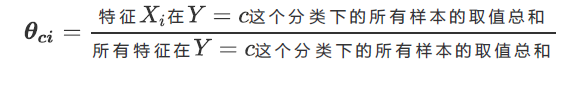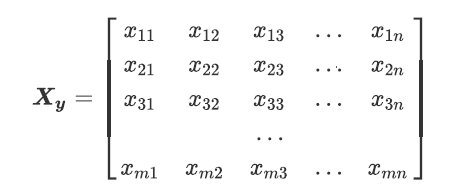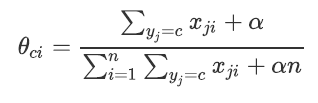class sklearn.naive_bayes.MultinomialNB (alpha=1.0, fit_prior=True, class_prior=None)
 参数 alpha : 浮点数, 可不填 (默认为1.0) 拉普拉斯或利德斯通平滑的参数，如果设置为0则表示完全没有平滑选项。但是需要注意的是，平滑相当于人 为给概率加上一些噪音，因此设置得越大，多项式朴素贝叶斯的精确性会越低（虽然影响不是非常大），布里 尔分数也会逐渐升高。 fit_prior : 布尔值, 可不填 (默认为True) 是否学习先验概率。如果设置为false，则不使用先验概率，而使用统一先验概率（uniform prior），即认为每个标签类出现的概率是。 class_prior：形似数组的结构，结构为(n_classes, )，可不填（默认为None） 类的先验概率。如果没有给出具体的先验概率则自动根据数据来进行计算。

from sklearn.preprocessing import MinMaxScaler
from sklearn.naive_bayes import MultinomialNB
from sklearn.model_selection import train_test_split
from sklearn.datasets import make_blobs
from sklearn.metrics import brier_score_loss

class_1=500
class_2=500
centers=[[0,0],[2,2]]# 设置数据集的中心
clusters_std=[0.5,0.5]# 设置两个类别的均值和方差
X,y=make_blobs(n_samples=[class_1,class_2],centers=centers,cluster_std=clusters_std
,random_state=0,shuffle=False)

xtrain,xtest,ytrain,ytest=train_test_split(X,y,random_state=0,test_size=0.3)

# 对数据进行归一化处理，防止出现负数
mms=MinMaxScaler().fit(xtrain)
xtrain_=mms.transform(xtrain)
xtest_=mms.transform(xtest)

# 现在实例化模型，进行拟合
# 训练的时候使用归一化之后的特征矩阵
mnb=MultinomialNB().fit(xtrain_,ytrain)

# 获取每一个特征的先验概率
# 返回每一个标签类对应的先验概率
mnb.class_log_prior_

mnb.class_log_prior_.shape
# 形状永远等于标签中所带的类别数量

# 返回每一个固定类别标签下的每一个特征的对数概率log(p(xi|y))
mnb.feature_log_prob_
# 矩阵形状是2*2,因为有2个特征，2个类别

# 在fit时每一个标签类别下包含的样本数
# 当fit时候接口中sample_weight被设置的时候，该接口返回的值也会受到加权的影响
mnb.class_count_
# 返回和我们标签类别一样的结构

mnb.predict(xtest)
# 返回我们的预测结果

# 返回每一个样本在每一个标签下面的取值概率
mnb.predict_proba(xtest)

# 返回我们的准确度
mnb.score(xtest,ytest)

brier_score_loss(ytest,mnb.predict_proba(xtest)[:,1],pos_label=1)
# 返回我们的布里尔分数
• 把连续性变量转换为离散型变量
#来试试看把Xtiain转换成分类型数据吧
#注意我们的Xtrain没有经过归一化，因为做哑变量之后自然所有的数据就不会又负数了
from sklearn.preprocessing import KBinsDiscretizer
kbs = KBinsDiscretizer(n_bins=10, encode='onehot').fit(Xtrain)
Xtrain_ = kbs.transform(Xtrain)
Xtest_ = kbs.transform(Xtest)
mnb = MultinomialNB().fit(Xtrain_, Ytrain)
mnb.score(Xtest_,Ytest)
brier_score_loss(Ytest,mnb.predict_proba(Xtest_)[:,1],pos_label=1)

### 3.2，伯努利朴素贝叶斯BernoulliNB

class sklearn.naive_bayes.BernoulliNB (alpha=1.0, binarize=0.0, fit_prior=True, class_prior=None)

 alpha : 浮点数, 可不填 (默认为1.0) 拉普拉斯或利德斯通平滑的参数，如果设置为0则表示完全没有平滑选项。但是需要注意的是，平滑相当于人 为给概率加上一些噪音，因此设置得越大，多项式朴素贝叶斯的精确性会越低（虽然影响不是非常大），布里 尔分数也会逐渐升高。 binarize : 浮点数或None，可不填，默认为0 将特征二值化的阈值，如果设定为None，则会假定说特征已经被二值化完毕 fit_prior : 布尔值, 可不填 (默认为True) 是否学习先验概率。如果设置为false，则不使用先验概率，而使用统一先验概率（uniform prior），即认为每个标签类出现的概率 class_prior：形似数组的结构，结构为(n_classes, )，可不填（默认为None） 类的先验概率。如果没有给出具体的先验概率则自动根据数据来进行计算。
# 使用伯努利贝叶斯或者多项式贝叶斯，一定要注意我们的数据结构
from sklearn.naive_bayes import MultinomialNB, GaussianNB, BernoulliNB
from sklearn.model_selection import train_test_split
from sklearn.datasets import make_blobs
from sklearn.preprocessing import KBinsDiscretizer
from sklearn.metrics import brier_score_loss as BS,recall_score,roc_auc_score as AUC

# 处理二项分布的朴素贝叶斯----伯努利朴素贝叶斯
from sklearn.naive_bayes import BernoulliNB
# 接下来进行数据的归一化
mms=MinMaxScaler().fit(xtrain)
xtrain__=mms.transform(xtrain)
xtest__=mms.transform(xtest)
# 先将数值全部进行归一化后，然后设置一个阈值，将数值进行二值化
# 先不设置二值化
bnl=BernoulliNB().fit(xtrain__,ytrain)
bnl.score(xtest__,ytest)
brier_score_loss(ytest,bnl.predict_proba(xtest__)[:,1],pos_label=1)
# 现在设置二值化的阈值
bnl=BernoulliNB(binarize=0.5).fit(xtrain__,ytrain)
bnl.score(xtest__,ytest)

### 3.3，探索贝叶斯：贝叶斯的样本不均衡问题

# 查看所有贝叶斯样本不均衡的表现
name = ["Multinomial","Gaussian","Bernoulli"]
models = [MultinomialNB(),GaussianNB(),BernoulliNB()]
for name,clf in zip(name,models):
Xtrain, Xtest, Ytrain, Ytest = train_test_split(X,y
,test_size=0.3
,random_state=420)
if name != "Gaussian":
kbs = KBinsDiscretizer(n_bins=10, encode='onehot').fit(Xtrain)
Xtrain = kbs.transform(Xtrain)
Xtest = kbs.transform(Xtest)
clf.fit(Xtrain,Ytrain)
y_pred = clf.predict(Xtest)
proba = clf.predict_proba(Xtest)[:,1]
score = clf.score(Xtest,Ytest)
print(name)
print("\tBrier:{:.3f}".format(BS(Ytest,proba,pos_label=1)))
print("\tAccuracy:{:.3f}".format(score))
print("\tRecall:{:.3f}".format(recall_score(Ytest,y_pred)))
print("\tAUC:{:.3f}".format(AUC(Ytest,proba)))
# 在这里解释一下：recall返佣的是少数类判断正确的比例

### 3.4，改进多项式朴素贝叶斯：补集朴素贝叶斯ComplementNB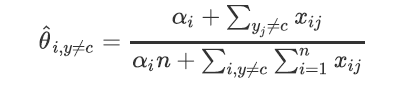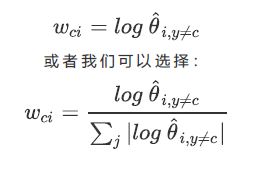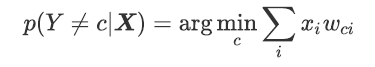class sklearn.naive_bayes.ComplementNB (alpha=1.0, fit_prior=True, class_prior=None, norm=False)
 alpha : 浮点数, 可不填 (默认为1.0) 拉普拉斯或利德斯通平滑的参数，如果设置为0则表示完全没有平滑选项。但是需要注意的是，平滑相当于人 为给概率加上一些噪音，因此设置得越大，多项式朴素贝叶斯的精确性会越低（虽然影响不是非常大），布里 尔分数也会逐渐升高。 norm : 布尔值，可不填，默认False 在计算权重的时候是否适用L2范式来规范权重的大小。默认不进行规范，即不跟从补集朴素贝叶斯算法的全部 内容，如果希望进行规范，请设置为True。 fit_prior : 布尔值, 可不填 (默认为True) 是否学习先验概率。如果设置为false，则不使用先验概率，而使用统一先验概率（uniform prior），即认为每个标签类出现的概率是。 class_prior：形似数组的结构，结构为(n_classes, )，可不填（默认为None） 类的先验概率。如果没有给出具体的先验概率则自动根据数据来进行计算。
from sklearn.naive_bayes import ComplementNB
from time import time
import datetime
name = ["Multinomial","Gaussian","Bernoulli","Complement"]
models = [MultinomialNB(),GaussianNB(),BernoulliNB(),ComplementNB()]
for name,clf in zip(name,models):
times = time()
Xtrain, Xtest, Ytrain, Ytest = train_test_split(X,y
,test_size=0.3
,random_state=420)
#预处理
if name != "Gaussian":
kbs = KBinsDiscretizer(n_bins=10, encode='onehot').fit(Xtrain)
Xtrain = kbs.transform(Xtrain)
Xtest = kbs.transform(Xtest)
clf.fit(Xtrain,Ytrain)
y_pred = clf.predict(Xtest)
proba = clf.predict_proba(Xtest)[:,1]
score = clf.score(Xtest,Ytest)
print(name)
print("\tBrier:{:.3f}".format(BS(Ytest,proba,pos_label=1)))
print("\tAccuracy:{:.3f}".format(score))
print("\tRecall:{:.3f}".format(recall_score(Ytest,y_pred)))
print("\tAUC:{:.3f}".format(AUC(Ytest,proba)))
print(datetime.datetime.fromtimestamp(time()-times).strftime("%M:%S:%f"))

10-14
08-08752712-19930
12-174647
03-202155
01-042万+
11-08230
02-131万+
11-25410
03-101318